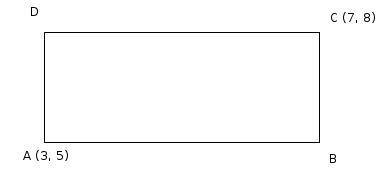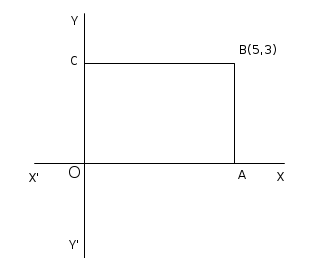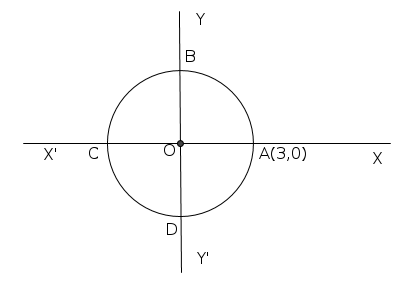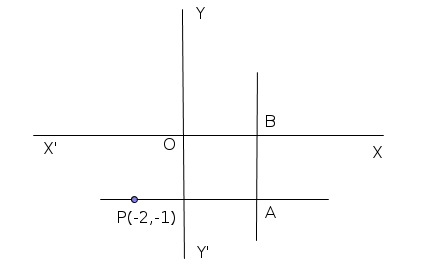Question Pool Coordinates

# Coordinates - SAMAGRA Question Pool & Answers | Class 10 English Medium

Kerala Syllabus SAMAGRA SCERT SAMAGRA Question Pool for Class 10 English Medium Maths CoordinatesQn 1.

Draw the X and Y axes, plot the points (0, 0), (0, 5), (5, 5), (5, 0) . Join the points in order. Write the suitable name for the quadrilateral.

To drawX and Y

Get Free Study Materials + 1 Week Free Trial of BrainsPrep Class 10 English Medium Tuition

Qn 2.In the figure ABCD is a rectangle and AB is parallel to the X-axis. Write the coordinates of B and D

Identify  the sides AD and BC are parallel to  Y axis

B (7, 5) D (3,

Get Free Study Materials + 1 Week Free Trial of BrainsPrep Class 10 English Medium Tuition

Qn 3.In the figure OABC is a rectangle. Write the coordinates of O, A and C.

O (0,

Get Free Study Materials + 1 Week Free Trial of BrainsPrep Class 10 English Medium Tuition

Qn 4.

Draw X, Y axes and mark the points A (1, 1), B (4, 1), C (4, 4), D (1, 4). What is the most suitable name of the figure obtained by joining these points in order.

Get Free Study Materials + 1 Week Free Trial of BrainsPrep Class 10 English Medium Tuition

Qn 5.

Centre of a circle is (2, 3) and radius is 7 units. Examine whether the point (8, 2) is on the circle .

Get Free Study Materials + 1 Week Free Trial of BrainsPrep Class 10 English Medium Tuition

Qn 6.

Classify the following points as points on the x-axis, on the y-axis and not on the axes

(4, 0), (0, 7), (3, 2), (1/2, 0), (0, 3/4), (-2, √2)

The points on X axis are (4, 0), (1/2, 0)

The points on Y axis are (0, 7), (0,

Get Free Study Materials + 1 Week Free Trial of BrainsPrep Class 10 English Medium Tuition

Qn 7.

In the figure O is the centre  of the circle. If the coordinates of A are (3, 0), find the coordinates of B, C, and DB (0, 3)

C (-3, 0)

D (0, -3)

Get Free Study Materials + 1 Week Free Trial of BrainsPrep Class 10 English Medium Tuition

Qn 8.

The opposite vertices of a rectangle having sides parallel to the axes are

(-2, 3), (5, 6).

Find the coordinates of the other vertices

.i) (5, 3), (-2,

Get Free Study Materials + 1 Week Free Trial of BrainsPrep Class 10 English Medium Tuition

Qn 9.

Draw X, Y axes and mark the points (-3, -1), (2, -1), (3, 2), (-2, 2) . What is the most suitable name of the figure obtained by joining these points in order.

Get Free Study Materials + 1 Week Free Trial of BrainsPrep Class 10 English Medium Tuition

Qn 10.

P (7, 3), Q (-5, 3) are two points on a line

a) Write the coordinates of another point on PQ

b) Write the coordinates of two points on a line which is parallel to PQ

(6, 3), (5, 3), (4, 3),

Get Free Study Materials + 1 Week Free Trial of BrainsPrep Class 10 English Medium Tuition

Qn 11.

In the figure, O is the origin and co-ordinates of the point P is (-2, -1). PA is parallel to X axis and AB is parallel to Y axis. If PA = 5 units, Find the coordinates of A and BA (3, -1)

B (3,

Get Free Study Materials + 1 Week Free Trial of BrainsPrep Class 10 English Medium Tuition

Qn 12.

A (5, 2), B (5, 7) are two points on a line

a) Write the coordinates of another point on AB

b) Write the coordinates of two points on a line which is perpendicular to AB

(5, 3), (5, 1), (5, 0), ......

Get Free Study Materials + 1 Week Free Trial of BrainsPrep Class 10 English Medium Tuition